# Python Global: How to Use Global Variable in Python

In Python, a global keyword allows you to modify the variable outside of the current scope. In this post, you’ll learn about the global keyword, global variable, and when to use global keywords in Python.

## Python Global Keyword

The global keyword is used to create the global variable and make changes to a variable in a local context. The basic rules for a global keyword in Python are the following.

1. When we create a variable inside the function, it is local by default.
2. When we define a variable outside of the function, it’s global by default. You don’t have to use the global keyword.
3. We use the global keyword to read and write a global variable inside the function.
4. The use of a global keyword outside the function does not affect.

Let’s take the example of a Global Keyword.

```# app.py

def myLocalFunc():
global site
site = 'AppDividend'
myLocalFunc()
print(site)```

See the output below.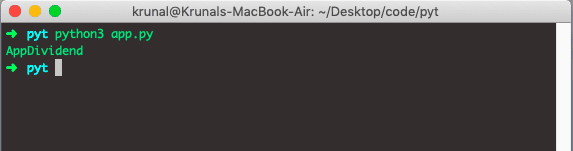In the above example, we have defined the Global variable inside the function. Then we have access that variable outside the function.

Due to we have defined the site variable as a Global, we can access that variable outside that function.

## Accessing global Variable From Inside a Function

Let’s take an example where we have defined one variable outside the function. Use that variable inside the function and see the output below.

```# app.py

site = 'AppDividend'

def myLocalFunc():
print(site)

myLocalFunc()```

See the output.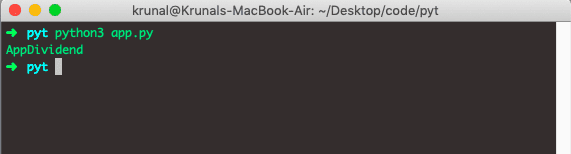## Modifying Global Variable From Inside the Function

In this example, we are defining one variable outside of the function and then change that variable inside the function and see the output of that variable.

```# app.py

site = 'AppDividend'

def myLocalFunc():
site = site + ': A Good Blog'
print(site)

myLocalFunc()```

Now, in the above example, we are trying to modifying that variable which is defined outside the function. Now, that function has no access to the outside variable. We will see an error in the output.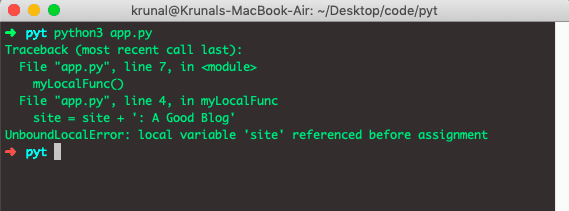## Changing Global Variable From Inside the Function using Global Keyword

Let’s take an example where we modify the Global variable inside the function using the Global keyword.

```# app.py

count = 21

def myLocalFunc():
global count
count = count - 2
print(count)

myLocalFunc()
print(count)```

The above program outputs 19 because we are modifying the global variable directly using the global keyword.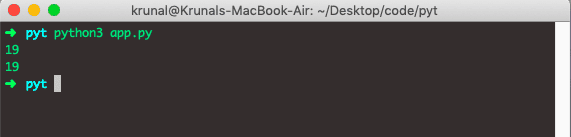## Global Variables Across Python Modules

In Python, we create a single module called shared.py to hold the global variables and share information across Python modules within the same program. Let’s take an example.

Now, first in this example, we will need three files.

1. shared.py
2. app.py
3. main.py

Write the following code inside the shared.py file.

```# shared.py

count = 21```

In this file, we have defined the global variable called count and assign the value 21.

Now, write the following code inside the app.py file.

```# app.py

import shared

shared.count = 19```

In this file, we have imported the shared file module and modify that variable to 19.

Finally, write the following code inside the main.py file.

```# main.py

import shared
import app

print(shared.count)```

Run the file by the following command.

`python3 main.py`

See the output below.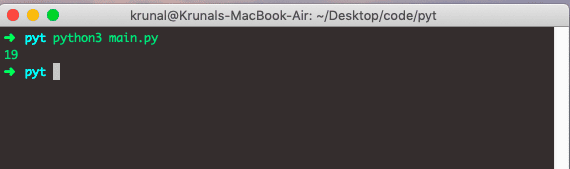The app.py modifies the global variable, and we have seen in the output.

So, we have seen almost all the use cases of Global keyword in Python.

That is it for Python global keyword.

### 1 thought on “Python Global: How to Use Global Variable in Python”

1.Thanks for the guide